# Engineering Mechanics - Force Vectors

### Exercise :: Force Vectors - General Questions

• Force Vectors - General Questions
26.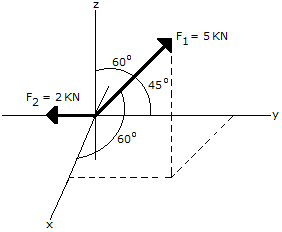Express each force in Cartesian vector form.

 A. F1 = (2.50 i + 3.54 j + 2.50 k) kN, F2 = -2 j kN B. F1 = (4.33 i + 3.54 j + 4.33 k) kN, F2 = -2 j kN C. F1 = (2.17 i + 3.75 j + 4.33 k) kN, F2 = -2 j kN D. F1 = (4.33 i + 3.75 j + 2.17 k) kN, F2 = -2 j kN

Explanation:

No answer description available for this question. Let us discuss.

27.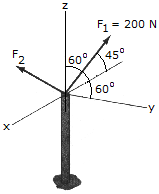Determine the magnitude and direction angles of F2, so that the resultant of the two forces acts upward along the z axis of the pole and has a magnitude of 275 N.

 A. F2 = 246 N,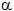= 54.9°,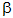= 114.0°,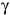= 44.7° B. F2 = 200 N,= 45.0°,= -60.0°,= 29.0° C. F2 = 200 N,= 45.0°,= 120.0°,= 29.0° D. F2 = 246 N,= 54.9°,= 66.0°,= 44.7°

Explanation:

No answer description available for this question. Let us discuss.

28.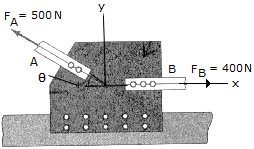Determine the design anglefor connecting member A to the plate if the resultant force is to be directed vercially upward. Also, what is the magnitude of the resultant?

 A. R = 400 N,= 53.5° B. R = 250 N,= 30.0° C. R = 300 N,= 36.9° D. R = 640 N,= 38.6°